Click to Chat

1800-1023-196

+91-120-4616500

CART 0

• 0

MY CART (5)

Use Coupon: CART20 and get 20% off on all online Study Material

ITEM
DETAILS
MRP
DISCOUNT
FINAL PRICE
Total Price: Rs.

There are no items in this cart.
Continue Shopping• Complete Physics Course - Class 11
• OFFERED PRICE: Rs. 2,968
• View Details

Thermodynamic Change or Thermodynamic Process

Table of Content

Isothermal Process

Isobaric Process

Isochoric Process

Quasi-static Process

Cyclic Process

Free Expansion

Solved Problems

Related ResourcesThermodynamic state of a system is characterized by its parameters P,V and T. A change in one or more parameters results in a change in the state of the system.

A process by which one or more parameters of thermodynamic system undergo a change is called a thermodynamic process or a thermodynamic change. Following are the various types of thermodynamic process.

(a) Isothermal Process

The process in which change in pressure and volume takes place at a constant temperature, is called a isothermal change. It may be noted that in such a change total amount of heat of the system does not remain constant.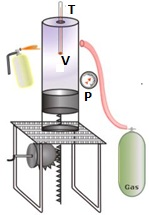Consider some gas contained in a barrel of conducting material, fitted with an airtight piston. As the gas is compressed as shown in the below figure, heat is liberated.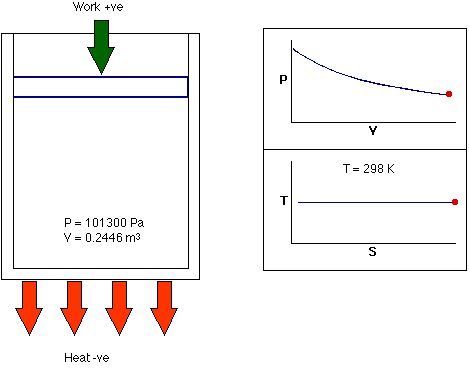The liberated heat moves out to keep the temperature constant. When the gas is expanded as shown in the below figure, heat is absorbed. This absorption is compensated by the transfer of heat from the surroundings, again keeping the temperature constant. Such a change is called an isothermal change. P-V diagram for an isothermal change is shown in below figure. The curve is called an ‘isothermal’.

It may be noted that in such a change total amount of heat of the system does not remain constant.

Since temperature remains constant, Boyle’s law can be applied to study this change.

Therefore, the equation of state for an isothermal change is given by,

PV=Constant

If P1, V1, and P2, V2 are the parameters of system in two different states, then for an isothermal change,

P1V1 = P2V2 = Constant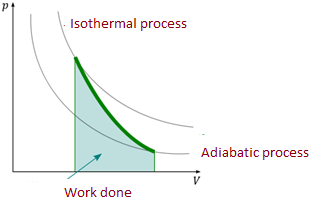The process in which change in pressure and volume and temperature takes place without any heat entering or leaving the system is called adiabatic change.

Consider some gas contained in an insulating barrel fitted with an air tight piston. On compressing the gas, heat is liberated. This liberated heat cannot move out due to the insulation of the barrel, resulting in a rise of temperature. On expanding the gas, heat is absorbed. This results in a fall of temperature. P-V diagram for an adiabatic change is shown in below figure.

It may be noted that the total heat of the system, undergoing an adiabatic change always remains constant.

The equation of state for adiabatic change is given by,

Where ‘’ is the ratio between the two specific heats of the gas.

Here Cp and Cv are the molar specific heat capacities of the gas at constant pressure and at constant volume respectively.

For the diatomic gases at medium temperatures (250 K to 750 K), ‘’ has a value of 1.42.

(c) Isobaric Process

The process in which change in volume and temperature of a gas take place at a constant pressure is called an isobaric process.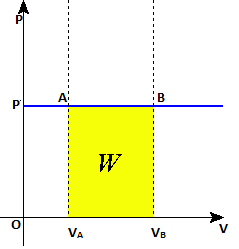Consider some gas contained in a barrel. Supply some quantity of heat to increase its temperature from T1 to T2 without changing its pressure ‘P’. It will be observed that the gas expand. The change is said to be isobaric change. P-V diagram for an isobaric change is a line parallel to V-axis as shown in below figure.

(d) Isochoric Process

The process in which changes in pressure and temperature take place in such a way that the volume of the system remains constant, is called isochoric process.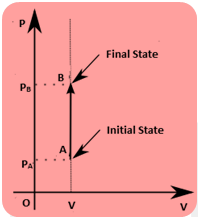Supply a quantity of heat Q to a gas contained in a barrel. The gas tends to expand. Let the expansion be prevented by increasing the pressure, on the piston, from ‘P’ to ‘P+p’, thus keeping its volume constant. As a result of this, the temperature of gas increases, P-V diagram for an isochoric change is a vertical line parallel to P-axis as shown in below figure.

(e) Quasi-static Process

The process in which change in any of the parameters take place at such a slow speed that the values of P,V, and T can be taken to be, practically, constant, is called a quasi-static process.(f) Cyclic Process

In a system in which the parameters acquire the original values, the process is called a cyclic process.

(g) Free Expansion

Such an expansion in which no external work is done and the total internal energy of the system remains constant is called free expansion.

Important Note:-

(a) Whenever change is said to take place suddenly, it should be taken as an adiabatic change. The word ‘suddenly’ implies that the system hardly finds time to get the heat exchanged with the surroundings.

(b) Conversely, if the change takes place slowly it should be considered as an isothermal change.

Solved Problems

Problem 1:-

In a motorcycle engine, after combustion occurs in the top of the cylinder, the piston is forced down as the mixture of gaseous products undergoes an adiabatic expansion. Find the average power involved in this expansion when the engine is running at 4000 rpm, assuming that the gauge pressure immediately after combustion is 1.50 atm, the initial volume is 50.0 cm3, and the volume of the mixture at the bottom of the stroke is 250 cm3. Assume that the gases are diatomic and that the time involved in the expansion is one-half that of the total cycle.

Concept:-

In an adiabatic process, the final pressure pf is defined as,

pf = piViγ/Vfγ

= pi [Vi/Vf]γ

The work done W for adiabatic process is defined as,

W = [pfVf - piVi]/(γ-1)

Here, initial pressure is pi, initial volume is Vi and final volume is Vf and adiabatic constant is γ.

Solution:-

First we have to find out final pressure pf. To obtain pf, substitute 16.0 atm for pi, 50.0 cm3 for Vi, 250 cm3 for Vf and 1.40 for γ in the equation pf = pi [Vi/Vf]γ,

pf = pi [Vi/Vf]γ

=(16.0 atm) [50/250]1.40

= 1.68 atm

To obtain the work done W by the gas during the expansion, substitute 16.0 atm for pi, 50.0 cm3 for Vi,1.68 atm for pf, 250 cm3 for Vf and 1.40 for γ in the equation W = [pfVf - piVi]/(γ-1), we get,

W = [pfVf - piVi]/(γ-1)

= (16.0 atm) (50.0 cm3) – (1.68 atm) (250 cm3)/(1.40-1)

= [(16.0 atm×(1.01×105 Pa/1 atm)) (50.0 cm3×(10-6 m3/1 cm3))] –[ (1.68 atm×(1.01×105 Pa/1 atm)) (250 cm3×(10-6 m3/1 cm3))]/(1.40-1)

=96.0 Pa.m3

= (96.0 Pa.m3) (1 N/m2/ 1 Pa)

= (96.0 N.m) (1 J/ 1 N.m)

= 96.0 J

The above process happens 4000 times per minute, but the actual time to complete the process is half of the cycle, or 1/8000 of a minute.

Thus the average power P involved in this expansion when the engine is running at 4000 rpm will be,

P = (96.0 J) (8000)/(60 s)

= 12.8×103 J/s

= (12.8×103 J/s) (1 W/1 J/s)

= 12.8×103 W

From the above observation we conclude that, the average power P involved in this expansion when the engine is running at 4000 rpm would be 12.8×103 W.

______________________________________________________________________________________________

Problem 2:-

A sample of gas expands from 1.0 to 5.0 m3 while its pressure decreases from 15 to 5.0 Pa. How much work is done on the gas if its pressure changes with volume according to each of the three process shown in the pV diagram in below figure.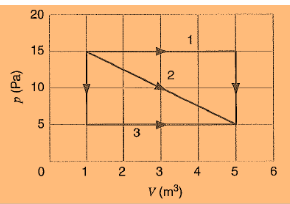Concept:-

Work is a path function. So work done on the gas depends upon the path. The area under pv diagram gives the work done on the gas between initial state i and final state f.

Work done W is defined as,

W = -pdV

Here p is the pressure and dV is the change in volume.

Solution:-

(1) In the process 1, the work done W1 will be zero for vertical path.

So W1 = 0 J

The work done is present only in horizontal path.

Thus the work done W2 for horizontal path will be,

W2= -pdV     (negative sign is due to work is done on the gas)

= - (15 Pa) (4m3)

= (-60 Pa.m3) (1 J/1 Pa.m3)

= -60 J

Therefore the net work done W on the gas will be,

W = W1 + W2

= 0 J+(-60 J)

= -60 J

From the above observation we conclude that, the net work done on the gas would be -60 J.

(2) For the process 2, work done is negative of the area under the curve.

So the area A under the curve for process 2 will be,

A = ½ (15 Pa+5 Pa) (4m3)

= (40 Pa.m3) (1 J/1 Pa.m3) = 40 J

As for the process 2, work done is negative of the area under the curve; therefore the net work done on the gas will be -40 J.

(3) In the process 3, the work done W3 will be zero for vertical path.

So W3 = 0 J

The work done is present only in horizontal path.

Thus the work done W4 for horizontal path will be,

W4= -pdV     (negative sign is due to work is done on the gas)

= - (5 Pa) (4m3)

= (-20 Pa.m3) (1 J/1 Pa.m3) = -20 J

Therefore the net work done W on the gas will be,

W = W3 + W4

= 0 J+(-20 J) = -20 J

From the above observation we conclude that, the net work done on the gas would be -20 J.

__________________________________________________________________________________

Problem 3:-

Calculate the work done by an external agent in compressing 1.12 mol of oxygen from a volume of 22.4 L and 1.32 atm pressure of 15.3 L at the same temperature.

Concept:-

In an isothermal process the work done by an external agent in compressing gas from its initial volume (Vi = V1  ) to its final volume (Vf = V2) is,

W = -nRT ln Vf / Vi

= -nRT ln V2/ V1 …… (1)

In accordance to ideal gas equation,

PV = nRT        …… (2)

Here P is the pressure of the gas, V is the volume of the gas, n is the number of moles, r is the gas constant and T is the temperature of the gas.

At constant temperature if the gas compress from its initial pressure P1, intial volume V1 to its final pressure P2, final volume V2, then

P1 V1 = P2 V2 = nRT       …… (3)

To find work W in terms of P1 and V1, substitute P1 V1 for nRT in the equation W = -nRT ln V2 / V1 ,

W  = -nRT ln V2/ V1

= -P1 V1 ln V2/ V1

Solution:-

To obtain work done, substitute 1.32 atm for pressure P1, 22.4 L for initial volume V1 and 15.3 L for initial volume V2 in the equation W = -P1 V1 ln V2/ V1,

W = -P1 V1 ln V2/ V1

= -(1.32 atm) (1.01×105 Pa / 1 atm) (22.4 L) (10-3 m3 / 1 L)  ln ((15.3 L) (10-3 m3/1 L) /(22.4 L) (10-3 m3 / 1 L))

= (1.14×103 Pa. m3) ( 1 J/1 Pa. m3)

= 1.14×103 J

From the above observation we conclude that, the work done by an external agent in compressing the oxygen molecule would be 1.14×103 J.

______________________________________________________________________________________________________

Question:-

Discuss the similarities and especially the distinctions between heat, work, and internal energy.

Heat:- It is the agent which produces in us the sensation of warmth and makes bodies hot. It is a form of energy. It flows from higher to lower temperature. In any exchange of heat, heat lost by the body is equal to the heat gained by the cold body. Substances generally expand when heated but the weight remains the same. A certain amount of heat known as latent heat is required to change the state of a body from solid to liquid or from liquid to gas without any change in temperature.

Internal energy:- Heat is not same as internal energy. Energy possessed by the atoms or molecules by virtue of their motion is called kinetic energy. There is a force of attraction between any two molecules; so they possess some potential energy. Sum of total kinetic and potential energies of atoms or molecules constituting a system are called the internal energy of the system. Thus heat is the part of internal energy which is transferred from one body to another an account of the temperature difference. Therefore the internal energy of a body was acquired by heat transfer.

If the internal energy of the system increases, then the sign of change in internal energy of the system is taken as positive and if the internal energy of the system decreases, then the sign of change in internal energy of the system is taken as negative.

Work: - Work is said to be done when force acting on a system displaces the body in its own direction. Work done W done on or by a system is the dot product of force and displacement. If the gas expands, work is said to be done by the system and the sign of work done is taken as positive. If the gas is compressed, work is said to be done on the system and the sign of work done is taken as negative.

Related Resources

You might like to refer  Second Law of Thermodynamics
For getting an idea of the type of questions asked, refer the Sample Papers of Previous Years.

To read more, Buy study materials of Thermodynamics comprising study notes, revision notes, video lectures, previous year solved questions etc. Also browse for more study materials on Chemistry here.### Course Features

• 101 Video Lectures
• Revision Notes
• Previous Year Papers
• Mind Map
• Study Planner
• NCERT Solutions
• Discussion Forum
• Test paper with Video Solution